## Saturday, February 16, 2013

### RTE.2.16Figure 1  Non Fixed EdgesFigure 2 Fixed Edges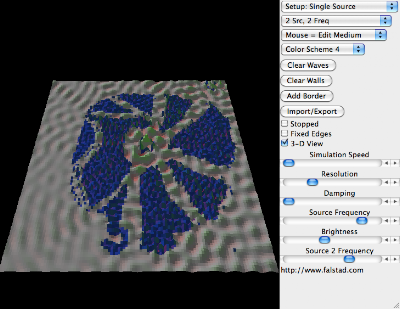Figure 3 Non Fixed Edges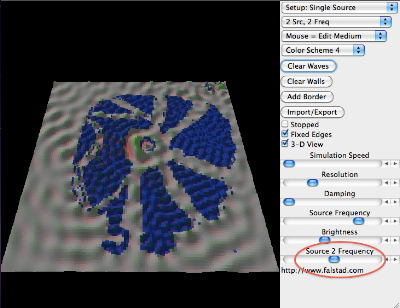Figure 4 Frequency Change Fixed EdgesFigure 5Non fixed edgesTime XFigure 4 BNon fixed edges Time X + .25 seconds

The point is at one relationship between frequencies the change from fixed to non fixed edges has little effect. With a small change in one frequency the effect is large.

## Thursday, February 14, 2013

### RTE.2.14Set Up While lines are viscosity boundariesFigure 1 2D both oscillators set at same frequencyFigure 1 3d Vieww

Figure 2 - Upper oscillator set to greater frequency than center,Figure 2 - 3d viewFigure 3 Coherence with non fixed edges Dif time Figure  2 aboveFigure 4 coherence by Fixed Edges.  Change made to Figure 1.

## Tuesday, February 12, 2013

### RTE.2.12.2Set up + 2 minutes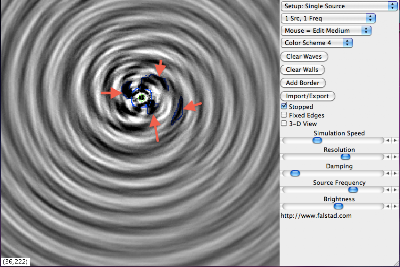Figure 2. Addition of viscosity lines based on peaks of pattern formation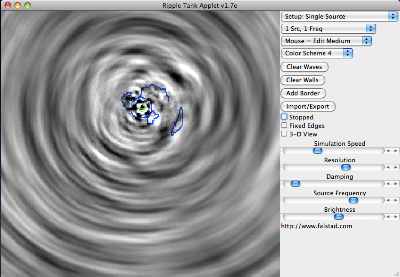Figure 2 +time X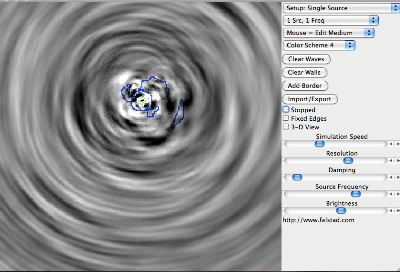Figure 4 - Fig 2 at X + Y timeFigure 5 - Figure 2 at  X + Y + Z time

## Monday, February 11, 2013

### RTE 2.11 Conversation

A conversation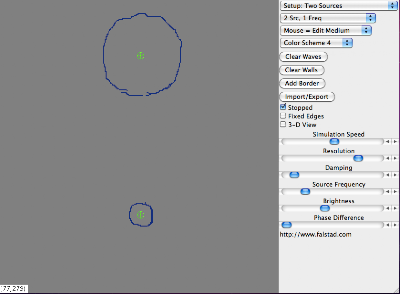Set up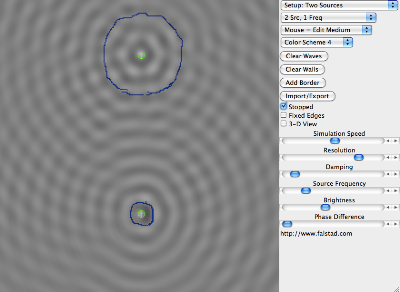Figure 1 at 30 secs Frequency Level oneFigure 2 at  60 seconds Frequency Level two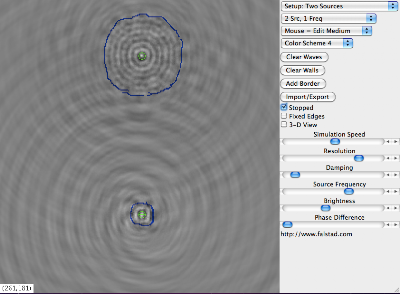Figure 3 at 90 seconds Frequency Level threeFigure 4 at  120 seconds Frequency Level four.